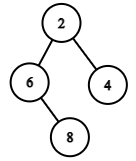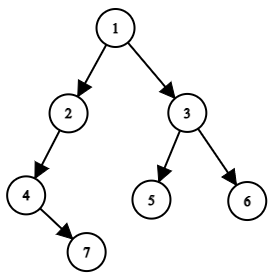# Print Binary Tree

Posted: 20 Mar, 2021
Difficulty: Moderate

## PROBLEM STATEMENT

#### If the parent node is placed at matrix[ row ][ column ], this cell will divide the rest space into two parts, i.e., left part and right part. You should print the left subtree of this parent node in the left part and the right subtree in the right part.

##### Note
``````If one of the left and right subtree is empty while the other is not, then for the empty part, you don’t have to print anything but have to leave the space as that of the nonempty size subtree.
``````
##### For Example
``````For the given binary tree
````````````Matrix will look like as

[ “ “,  “ “,  “ “,  “2”,  “ “,  “ “,  “ “ ]
[ “ “,  “6 “,  “ “,  “ “,  “ “,  “4 “,  “ “ ]
[ “ “,  “ “,  “8 “,  “ “,  “ “,  “ “,  “ “ ]
``````
##### Input Format
``````The first line contains an integer 'T' which denotes the number of test cases. Then the test cases are as follows.

The first line of each test case contains elements of the tree in the level order form. The line consists of values of nodes separated by a single space. In case a node is null, we take -1 in its place.
````````````For example, the input for the tree depicted in the above image would be :

1
2 3
4 -1 5 6
-1 7 -1 -1 -1 -1
-1 -1
``````

#### Explanation :

``````Level 1 :
The root node of the tree is 1

Level 2 :
Left child of 1 = 2
Right child of 1 = 3

Level 3 :
Left child of 2 = 4
Right child of 2 = null (-1)
Left child of 3 = 5
Right child of 3 = 6

Level 4 :
Left child of 4 = null (-1)
Right child of 4 = 7
Left child of 5 = null (-1)
Right child of 5 = null (-1)
Left child of 6 = null (-1)
Right child of 6 = null (-1)

Level 5 :
Left child of 7 = null (-1)
Right child of 7 = null (-1)
``````
##### Note :
``````The above format was just to provide clarity on how the input is formed for a given tree.
The sequence will be put together in a single line separated by a single space. Hence, for the above-depicted tree, the input will be given as:

1 2 3 4 -1 5 6 -1 7 -1 -1 -1 -1 -1 -1
``````
##### Output Format
``````For each test, print the binary tree in 2-D array of strings.

Print the output of each test case in a separate line.
``````
##### Note:
``````You do not need to print anything, it has already been taken care of. Just implement the given function.
``````
##### Constraints:
``````1 <= T <= 50
0 <= N <= 10^3
1 <= data <= 10^4

Where “data” is the value of the binary tree node.

Time Limit: 1 sec
``````Approach 1

We will recursively print every node value in the matrix. Before that, we have to keep some points in mind -

• The number of rows in the resultant matrix = height of the tree.
• The number of columns in the resulting matrix = 2^height - 1.
• If the parent node is in the middle of ‘left’ and ‘right’, let’s say ‘ mid. ‘  then its left child will be placed at the center of the left and mid, and its right child is placed at the center of mid + 1 and right.
• Take a 2-D array of string  ‘matrix’ of size height x (2^height - 1 ) and initialize all values with an empty string “ ”.
• We will make a recursive function printTree( root, matrix, row, left, right) where ‘row’ denotes the row in which the current element has to be placed, left and right are the columns’ indices in the middle of which the current element is placed.
• Current element will be placed at matrix[ row ][ mid ] where mid = ( left + right ) / 2.
• The left child will be placed in the next row, and column = middle of the left and mid, so we will recursively call printTree( root->left, matrix, row +1, left, mid).
• Similarly, for right child recursively call printTree(root->right,  matrix, row + 1, mid +1, right ).

Algorithm:

1. Let printTree( ROOT, MATRIX, ROW, LEFT, RIGHT ) be our recursive function.
2. If ‘ROOT’ = NULL return ‘MATRIX’.
3. Take a variable ‘MID’ to find the center of the left and right indices.
4. Put current node’s data at MATRIX[ ROW ][ MID ].
5. Recursively call  printTree( ROOT -> left, MATRIX, ROW + 1, LEFT, MID )
6. Recursively call  printTree( ROOT -> right, MATRIX, ROW + 1, MID + 1, RIGHT ).
7. Return ‘MATRIX.’